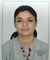# New to Qlik Sense

If you’re new to Qlik Sense, start with this Discussion Board and get up-to-speed quickly.

Announcements
Welcome to Qlik Community! Check out our new navigation! FIND OUT MORE
cancel
Showing results for
Did you mean:Contributor

## Percentage change between to expressions

Hi,

i am  trying to find a formula for a KPI where two expressions get compared and  the output should be in percentage.

I used to do this with:

=((Sum({\$<Arbeitspl.={0101}>}[Menge (ER)]))

/

(Sum({\$<Platz={'S1'}>}[WIP T1])))-1

This works fine for me, but if one of the numbers is Null ("0") it obviously doesn't.

Any ideas?

Labels (5)

• ### Percentage changes

1 Solution

Accepted SolutionsMaster II

Hi Peter,

May be try Alt function, as if your denominator become then you are expected to get null value. So you can use alt in such cases.

Alt(

((Sum({\$<Arbeitspl.={0101}>}[Menge (ER)]))

/

(Sum({\$<Platz={'S1'}>}[WIP T1])))-1

,'NA')

Best Regards,
KC
3 RepliesMaster II

Hi Peter,

May be try Alt function, as if your denominator become then you are expected to get null value. So you can use alt in such cases.

Alt(

((Sum({\$<Arbeitspl.={0101}>}[Menge (ER)]))

/

(Sum({\$<Platz={'S1'}>}[WIP T1])))-1

,'NA')

Best Regards,
KCCreator II

if i understood it right...

if(Sum({\$<Platz={'S1'}>}[WIP T1])=0,Sum({\$<Arbeitspl.={0101}>}[Menge (ER)]),((Sum({\$<Arbeitspl.={0101}>}[Menge (ER)]))

/

(Sum({\$<Platz={'S1'}>}[WIP T1])))-1)

Thanks,

PriyankaContributor
Author

Thank you,

it was a little different but i found a solution with your help!Tags
Community Browser